# Distance and Displacement

• Last Updated : 03 Feb, 2022

If an object changes its position with respect to time, the object is known to be in motion. The path for the motion shall vary, it is what defines the different types of motion. If the motion is only in one coordinate, it becomes 1-D or one-dimensional motion, if the motion is in two coordinates, it becomes 2-D or two-dimensional motion, if the motion includes all the three coordinates, it becomes a 3-D or three-dimensional motion. Motion is known to a relative, and it is explained through the frame of reference. Let’s start by learning what Frame of Reference is,

### Frame of Reference

Suppose two friends are standing on the opposite sides of the road and a vehicle is passing by them. Friends 1 realizes that the vehicle is passing by his right side while friend 2 realizes that the vehicle is passing by his left side. They observed two different situations for the same vehicle, this is because they have a different frame of reference. The frame of reference is generated by the reference points which is different in both of the friends’ cases. The reference points were the opposite sides of the roads in this case.

In Physics, there are three coordinates, and they are mutually perpendicular to each other. The co-ordinate geometry is actually the space in real life. The coordinates coincide with each other and where they do, the values of all the coordinates are at 0. It is called as the origin. The origin is the Reference point. Humans see the world with their eyes as the reference point and all the motion occurs relative to their position.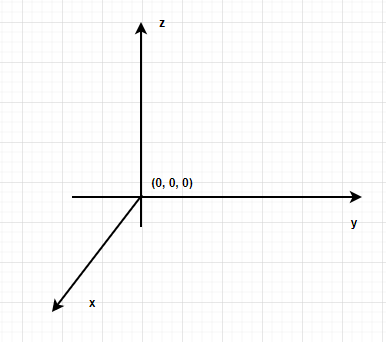### One-Dimensional Motion

One-dimensional motion or rectilinear motion is motion in a straight line, only covering one of the three coordinates. For example, a train moving on a straight track, motion under free fall.

Linear motion or rectilinear motion can be of two types,

Uniform Linear motion

Motion is known to be uniform when the body covers equal distance in equal amount of time moving on a straight line.

Non- uniform Linear motion

Motion is known to be non-uniform when the body covers unequal distance in equal amount of time moving on a straight line.

### Distance

Distance is the total path covered by the object in motion, irrespective of the direction of the path. Distance is known to be a scalar quantity as only path length covered is measured and the direction of motion is not taken into account. The distance can only be positive.

Distance gives detailed information of the entire path covered during motion. Distance is represented as ‘d’ and it is not represented with an arrow as the direction is not involved.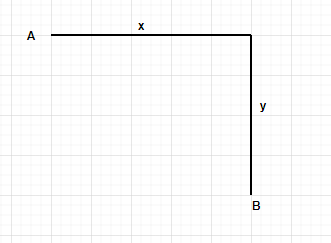Distance covered from A to B = (x+ y) units.

Formula for Distance (d) = Speed × Time

### Displacement

Displacement is defined as the total change in the position of the object along with the direction of motion. Displacement is known to be vector quantity as along with the magnitude of the changed position, the direction of the motion is also taken into account. Displacement can be positive, negative, or zero.

Displacement does not give detailed information of the entire path length, the initial and the final position is required for the calculation of the displacement. Displacement is represented as ‘S’ and an arrow is used to indicate that displacement is a vector quantity. Displacement always has a straight-lined path as the initial and final points are directly connected to find the displacement.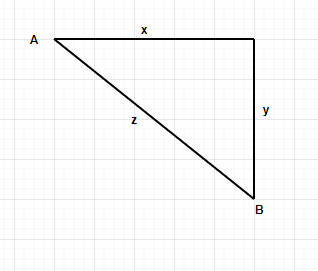Displacement from A to B= z units (in the south-east direction).

Formula for Displacement= velocity × time.

### Sample Problems

Question 1: List out at least 3 differences between distance and displacement.

Difference between distance and displacement,

Question 2: What is the distance and displacement from the given figure,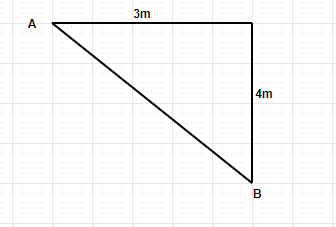Solution:

Distance is the total path length,

Distance = (3+ 4) m = 7meters.

Displacement is the direct line from A to B,

Displacement=Therefore, Displacement= 5 meters (south- east direction).

Question 3: A particle has covered a distance of 1000 meters and a displacement of 200 meters in 1minute 40 seconds. Find the Speed and Velocity of the particle during motion?

Solution:

Time taken = 1 minute 40 seconds = 100 seconds

Speed= distance/ time

Speed= 1000/ 100 = 10m/sec

Velocity= displacement/ time

Velocity= 200/100 = 2 m/sec

Question 4: A boy is facing North, and he starts moving, he walked 5 meters straight and then turns right and walked 3 meters, then he turns right and again walks 5 meters. What is the distance and displacement covered by the boy?

Solution:

The figure for the path traveled by the boy shall look like,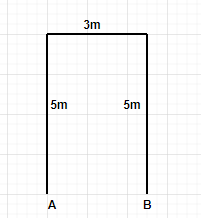Distance Covered by the boy= (5+ 3+ 5) m = 13m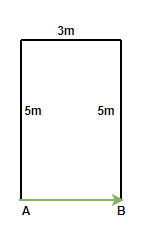Displacement= 3 m (in the east direction)

Question 5: The speed and the velocity of a car is given as 5 m/sec, and the time taken in the whole journey is 5 minutes, what are the observations drawn from that information?

Solution:

Time taken in the whole journey= 5 minutes= 5× 60= 300 seconds.

Speed given= 5 m/sec

Distance= 5× 300= 1500 meters= 1.5 km

Velocity= 5 m/sec

Displacement= 5× 300= 1500 meters= 1.5 km.

Observation- The distance and the displacement are both equal since the speed and the velocity of the car is equal. Therefore, it can be said that the car has traveled only in a straight direction.

Question 6: A boy decided to take a walk around his town in the evening, he started from his home and traveled approximately 1500 meters in 30 minutes. He finally came back to his home. Find the values of the following quantities,

• Speed
• Displacement
• Velocity

Solution:

Given: Distance= 1500 meters, Time= 30 minutes= 30× 60 seconds= 1800 seconds.

Speed = distance/ time

Speed= 1500/1800= 0.833 m/sec

Displacement= 0 meters (as the boy finally came back to his house, so the initial and the final point coincided).

Velocity = Displacement/ time= 0/ 1800= 0 m/sec

My Personal Notes arrow_drop_up